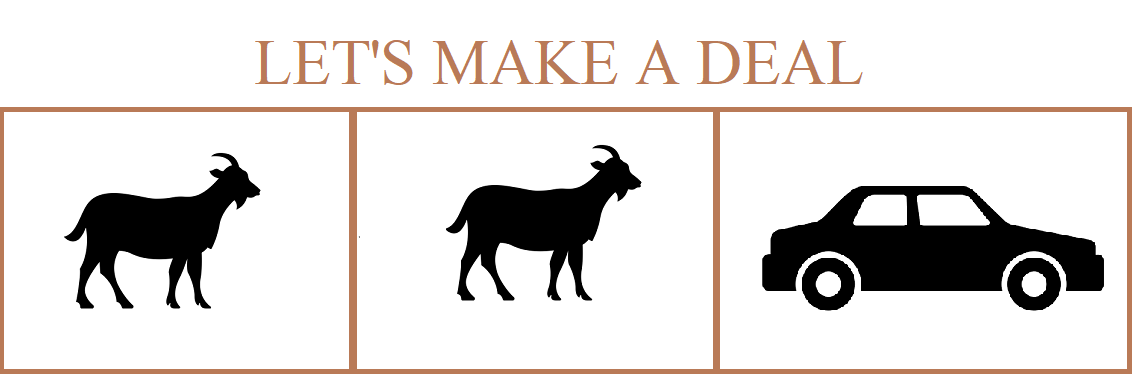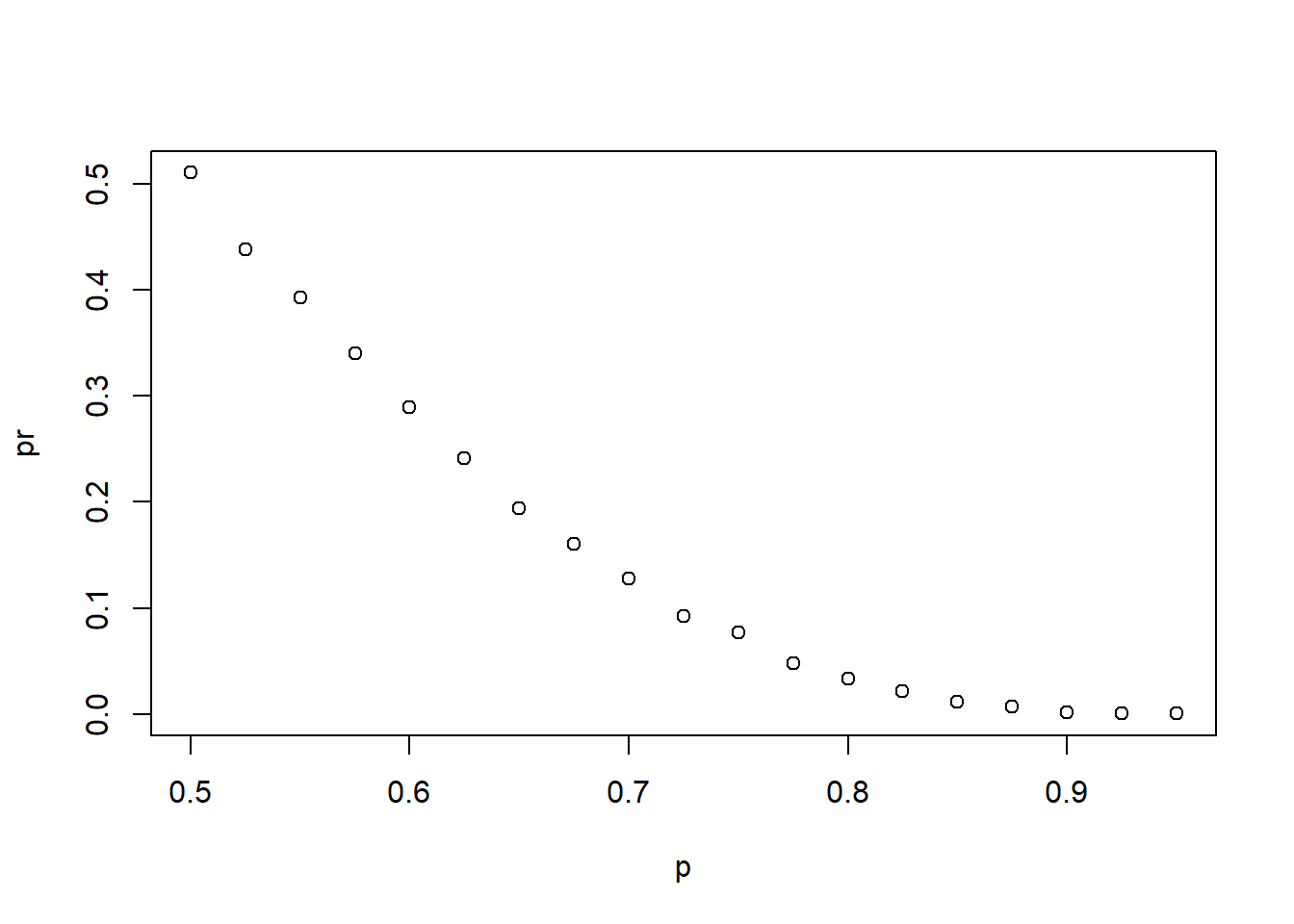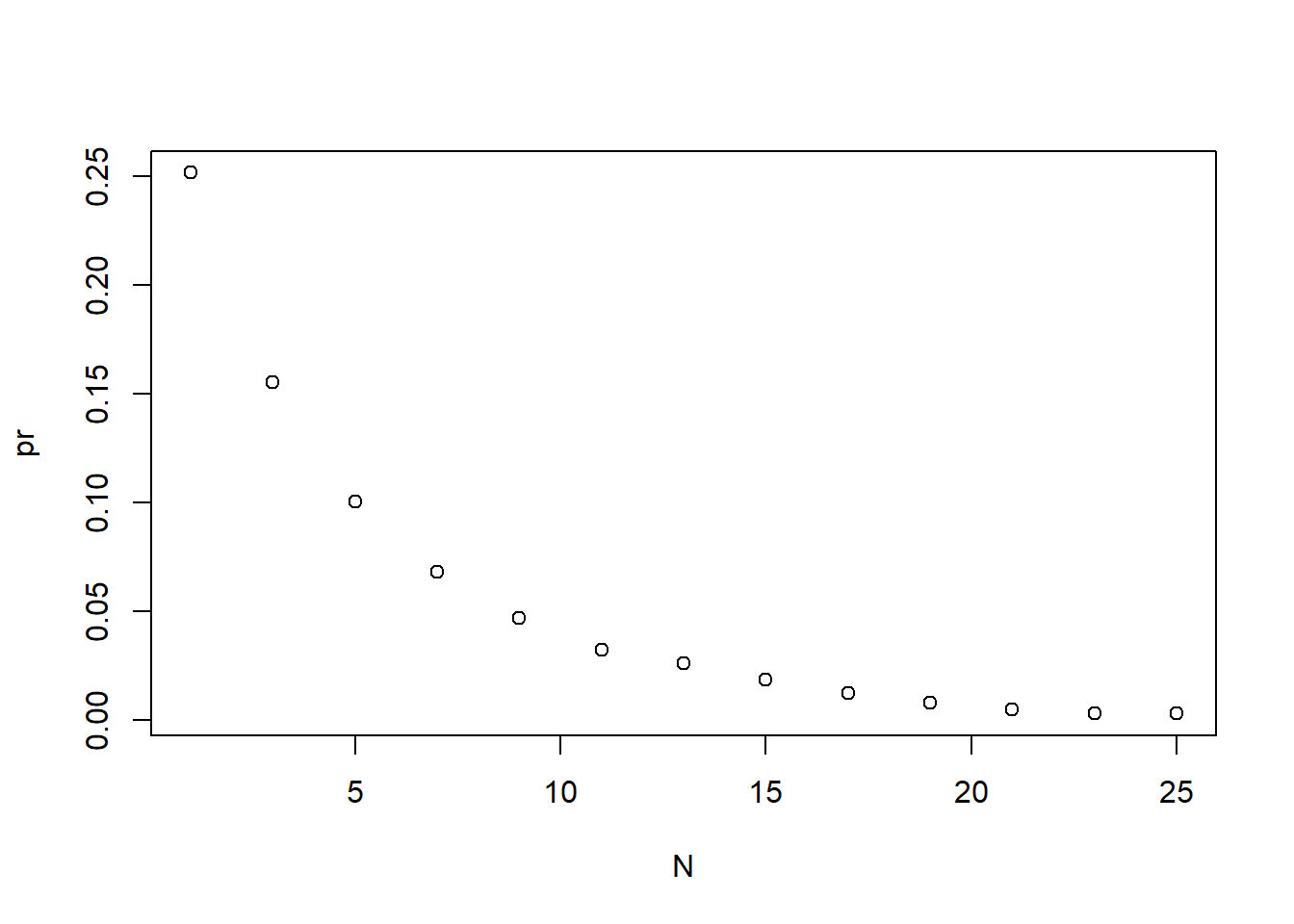l

The Monty Hall problem is a brain teaser, originated from the American television game show Let’s Make a Deal and named after its original host, Monty Hall. It is basically a probability puzzle, originally posed (and solved) in a letter by Steve Selvin to the American Statistician in 1975. It became famous as a question from reader Craig F. Whitaker’s letter quoted in Marilyn vos Savant’s “Ask Marilyn” column in Parade magazine in 1990.

The game host asked the contestants to pick one of three doors. Behind one door there was a prize. The other doors had a goat behind them to show the contestant they had lost. After the contestant picked a door, before revealing whether the chosen door contained a prize, Monty Hall would open one of the two remaining doors and show the contestant there was no prize behind that door. Then he would ask “Do you want to switch doors?” What would you do?

We can use probability to show that if you stick with the original door choice, your chances of winning a prize remain 1 in 3. However, if you switch to the other door, your chances of winning double to 2 in 3. This seems counter intuitive. Many people incorrectly think both chances are 1 in 2 since you are choosing between 2 options. We can use the following Monte Carlo simulation to see which strategy is better. Note that this code is written longer than it should be for pedagogical purposes.

B <- 10000
monty_hall <- function(strategy){
doors <- as.character(1:3)
prize <- sample(c("car", "goat", "goat"))
prize_door <- doors[prize == "car"]
my_pick  <- sample(doors, 1)
show <- sample(doors[!doors %in% c(my_pick, prize_door)],1)
stick <- my_pick
stick == prize_door
switch <- doors[!doors%in%c(my_pick, show)]
choice <- ifelse(strategy == "stick", stick, switch)
choice == prize_door
}
stick <- replicate(B, monty_hall("stick"))
mean(stick)
##  0.3292
switch <- replicate(B, monty_hall("switch"))
mean(switch)
##  0.6681

The analysis reveals that the chance is 1 in 3, what we began with. Now switching to the Monte Carlo simulation confirms the 2/3. Monte Carlo helps us to gain some insight by showing that we are removing a door, show, that is definitely not a winner from our choices. We also see that unless we get it right when we first pick, you win: 1 - 1/3 = 2/3.

Now I am presenting the following two problems that are related to Monty Hall problem that I picked up from the problems that I had to solve on various important topics on “Data Science” during my professional certification on “Data Science in R” with edX of the Harvard University. Please enjoy:

Example-1:

Two teams, say the Cavs and the Warriors, are playing a seven game championship series. The first to win four games, therefore, wins the series. The teams are equally good so they each have a 50-50 chance of winning each game. If the Cavs lose the first game, what is the probability that they win the series?

Solution:

# Assign a variable 'n' as the number of remaining games.
n <- 6

# Assign a variable outcomes as a vector of possible game outcomes, where 0 indicates a loss and 1 indicates a win for the Cavs.
outcomes <- c(0,1)

# Assign a variable lis to a list of all possible outcomes in all remaining games. Use the rep function on list(outcomes) to create list of length n.

lis <- rep(list(outcomes), n)

# Create a data frame named 'poss' that contains all combinations of possible outcomes for the remaining games.

poss <- expand.grid(lis)
# Create a vector named 'res' that indicates whether each row in the data frame 'poss' contains enough wins for the Cavs to win the series.

res <- rowSums(poss)>=4
# Calculate the proportion of 'res' in which the Cavs win the series. Print the outcome to the console.

mean(res)
##  0.34375

Example-2:

Confirm the results of the previous question with a Monte Carlo simulation.

Solution:

##  0.3422

Example-3:

Two teams, A and B, are playing a seven game series. Team A is better than team B and has a p > 0.5 chance of winning each game.

Given a value p, the probability of winning the series for the underdog team B can be computed with the following function based on a Monte Carlo simulation:

prob_win <- function(p){ B <- 10000 result <- replicate(B, { b_win <- sample(c(1,0), 7, replace = TRUE, prob = c(1-p, p)) sum(b_win)>=4 }) mean(result) }

Use the function sapply to compute the probability, call it Pr, of winning for p <- seq(0.5, 0.95, 0.025). Then plot the result.Example-4:

Repeat the exercise above, but now keep the probability fixed at p <- 0.75 and compute the probability for different series lengths: best of 1 game, 3 games, 5 games,… Specifically, N <- seq(1, 25, 2). Hint: use this function:

prob_win <- function(N, p=0.75){ B <- 10000 result <- replicate(B, { b_win <- sample(c(1,0), N, replace = TRUE, prob = c(1-p, p)) sum(b_win)>=(N+1)/2 }) mean(result) }

N <- seq(1, 25, 2)
prob_win <- function(N, p=0.75){
B <- 10000
result <- replicate(B, {
b_win <- sample(c(1,0), N, replace = TRUE, prob = c(1-p, p))
sum(b_win)>=(N+1)/2
})
mean(result)
}
pr <- sapply(N, prob_win)
plot(N,pr)